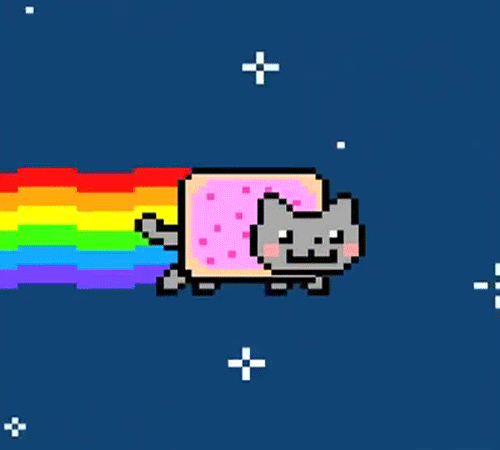# Creating GIFs in Python using Pillow (PIL Fork)

I was working on a personal project the other day and I needed to create some images (frames) and save them as a playable GIF. Working in Python, I excepted to find an easy solution fast but oh boy did it take me too long to find it.

Here I am now, creating a blog post to help future people looking to create gifs in Python.

The code used in this post is available here.

## What are GIFs

The **Graphics Interchange Format **is a bitmap image format that was developed in 1987.

A GIF is basically a series of images that have different settings such as:

• Looping
• Duration per frame (image)
• Others…

A GIF can also be a static image.An example of a GIF (Nyan Cat ♥)

## What is Pillow (PIL Fork)

Python Imaging Library (PIL) is a free library for the Python programming language that adds support for opening, manipulating, and saving many different image file formats.

On the other hand, Pillow is a fork of the PIL library introducing many changes and enhancements, whether to make the API easier to use or to handle more image formats and requirements.

N.B: PIL and Pillow cannot co-exist, you can only install/use one or the other.

## Creating GIFs in Python

### Installing Pillow

You can easily install Pillow using Pip:

``````pip install Pillow
``````

### Creating GIFs

As an example, I will be creating a GIF where a ball moves down.

At first, we’ll create a method that creates an image and draws a ball on it, this will help us keep things clean.

``````from PIL import Image, ImageDraw

def create_image_with_ball(width, height, ball_x, ball_y, ball_size):
img = Image.new('RGB', (width, height), (255, 255, 255))
draw = ImageDraw.Draw(img)
# draw.ellipse takes a 4-tuple (x0, y0, x1, y1) where (x0, y0) is the top-left bound of the box
# and (x1, y1) is the lower-right bound of the box.
draw.ellipse((ball_x, ball_y, ball_x + ball_size, ball_y + ball_size), fill='red')
return img
``````

In the code above, we create a new RGB image using `Image.new` that has a certain size and a white background.

We then use `ImageDraw` to draw a red ellipse (circle) into our image using the coordinates given in the arguments.

All we have to do now is create multiple images (frames) while moving the ball down:

``````# Create the frames
frames = []
x, y = 0, 0
for i in range(10):
new_frame = create_image_with_ball(400, 400, x, y, 40)
frames.append(new_frame)
x += 40
y += 40

# Save into a GIF file that loops forever
frames.save('moving_ball.gif', format='GIF', append_images=frames[1:], save_all=True, duration=100, loop=0)
``````

Here’s the breakdown of the code above:

1. Initialize an empty list `frames` and 0-coordinates `x` and `y`.
2. In a for loop (10 runs), we create a 400×400 image with a ball in our coordiantes.
3. Change the coordinates so that the ball moves down (in diagonal, since the Y position changes too).
4. Save the first frame with the others using `format='GIF'` and `append_images=frames[1:]`.
• Every frame will run for 100ms (`duration=100`).
• The GIF image will loop forever (`loop=0`, if loop was set to 1 then it will loop 1 time, 2 = 2 times, etc…).

Our magnificent moving ball looks like this:Moving ball

I hope this can help anyone looking for a way to handle images and create GIFs!

Zanid Haytam is an enthusiastic programmer that enjoys coding, reading code, hunting bugs and writing blog posts.

comments powered by Disqus